Home Practice
For learners and parents For teachers and schools
Textbooks
Full catalogue
Pricing SupportLog in

We think you are located in United States. Is this correct?

# 4.6 Electronic configuration

## 4.6 Electronic configuration (ESABE)

### The energy of electrons (ESABF)

The electrons of an atom all have the same charge and the same mass, but each electron has a different amount of energy. Electrons that have the lowest energy are found closest to the nucleus (where the attractive force of the positively charged nucleus is the greatest) and the electrons that have higher energy (and are able to overcome the attractive force of the nucleus) are found further away.

### Electron arrangement (ESABG)

We will start with a very simple view of the arrangement or configuration of electrons around an atom. This view simply states that electrons are arranged in energy levels (or shells) around the nucleus of an atom. These energy levels are numbered 1, 2, 3, etc. Electrons that are in the first energy level (energy level 1) are closest to the nucleus and will have the lowest energy. Electrons further away from the nucleus will have a higher energy.

In the following examples, the energy levels are shown as concentric circles around the central nucleus. The important thing to know for these diagrams is that the first energy level can hold 2 electrons, the second energy level can hold 8 electrons and the third energy level can hold 8 electrons.

1. Lithium

Lithium ($$\text{Li}$$) has an atomic number of 3, meaning that in a neutral atom, the number of electrons will also be 3. The first two electrons are found in the first energy level, while the third electron is found in the second energy level (Figure 4.7).

2. Fluorine

Fluorine ($$\text{F}$$) has an atomic number of 9, meaning that a neutral atom also has 9 electrons. The first 2 electrons are found in the first energy level, while the other 7 are found in the second energy level (Figure 4.8).

3. Neon

Neon ($$\text{Ne}$$) has an atomic number of 10, meaning that a neutral atom also has 10 electrons. The first 2 electrons are found in the first energy level and the last 8 are found in the second energy level. (Figure 4.9).

But the situation is slightly more complicated than this. Within each energy level, the electrons move in orbitals. An orbital defines the spaces or regions where electrons move.

Atomic orbital

An atomic orbital is the region in which an electron may be found around a single atom.

The first energy level contains only one s orbital, the second energy level contains one s orbital and three p orbitals and the third energy level contains one s orbital and three p orbitals (as well as five d orbitals). Within each energy level, the s orbital is at a lower energy than the p orbitals. This arrangement is shown in Figure 4.10.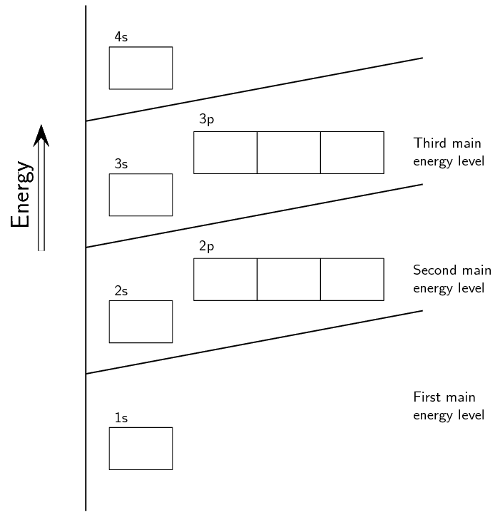Figure 4.10: The positions of the first ten orbitals of an atom on an energy diagram.

Each block in Figure 4.10 is able to hold two electrons. This means that the s orbital can hold two electrons, while the p orbital can hold a total of six electrons, two in each of the three blocks.

This diagram also helps us when we are working out the electron configuration of an element. The electron configuration of an element is the arrangement of the electrons in the shells and subshells. There are a few guidelines for working out the electron configuration. These are:

• Each orbital can only hold two electrons. Electrons that occur together in an orbital are called an electron pair.

• An electron will always try to enter an orbital with the lowest possible energy.

• An electron will occupy an orbital on its own, rather than share an orbital with another electron. An electron would also rather occupy a lower energy orbital with another electron, before occupying a higher energy orbital. In other words, within one energy level, electrons will fill an s orbital before starting to fill p orbitals.

• The s subshell can hold 2 electrons

• The p subshell can hold 6 electrons

The way that the electrons are arranged in an atom is called its electron configuration.

When there are two electrons in an orbital, the electrons are called an electron pair. If the orbital only has one electron, this electron is said to be an unpaired electron. Electron pairs are shown with arrows pointing in opposite directions.

Electron configuration

Electron configuration is the arrangement of electrons in an atom, molecule or other physical structure.

### Aufbau diagrams (ESABH)

An element's electron configuration can be represented using Aufbau diagrams or energy level diagrams. An Aufbau diagram uses arrows to represent electrons. You can use the following steps to help you to draw an Aufbau diagram:

1. Determine the number of electrons that the atom has.

2. Fill the s orbital in the first energy level (the 1s orbital) with the first two electrons.

3. Fill the s orbital in the second energy level (the 2s orbital) with the second two electrons.

4. Put one electron in each of the three p orbitals in the second energy level (the 2p orbitals) and then if there are still electrons remaining, go back and place a second electron in each of the 2p orbitals to complete the electron pairs.

5. Carry on in this way through each of the successive energy levels until all the electrons have been drawn.

You can think of Aufbau diagrams as being similar to people getting on a bus or a train. People will first sit in empty seats with empty seats between them and the other people (unless they know the people and then they will sit next to them). This is the lowest energy. When all the seats are filled like this, any more people that get on will be forced to sit next to someone. This is higher in energy. As the bus or train fills even more the people have to stand to fit on. This is the highest energy.

Aufbau is the German word for “building up”. Scientists used this term since this is exactly what we are doing when we work out electron configuration, we are building up the atoms structure.

#### Hund's rule and Pauli's principle

Sometimes people refer to Hund's rule for electron configuration. This rule simply says that electrons would rather be in a subshell on their own than share a subshell. This is why when you are filling the subshells you put one electron in each subshell and then go back and fill the subshell, before moving onto the next energy level.

Pauli's exclusion principle simply states that electrons have a property known as spin and that two electrons in a subshell will not spin the same way. This is why we draw electrons as one arrow pointing up and one arrow pointing down.

### Spectroscopic electron configuration notation (ESABI)

A special type of notation is used to show an atom's electron configuration. The notation describes the energy levels, orbitals and the number of electrons in each. For example, the electron configuration of lithium is $$1\text{s}^{2}2\text{s}^{1}$$. The number and letter describe the energy level and orbital and the number above the orbital shows how many electrons are in that orbital.

Aufbau diagrams for the elements fluorine and argon are shown in Figure 4.11 and Figure 4.12 respectively. Using spectroscopic notation, the electron configuration of fluorine is $$1\text{s}^{2}2\text{s}^{2}2\text{p}^{5}$$ and the electron configuration of argon is $$1\text{s}^{2}2\text{s}^{2}2\text{p}^{6}3\text{s}^{2}3\text{p}^{6}$$.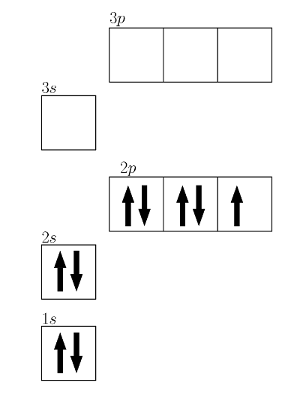Figure 4.11: An Aufbau diagram showing the electron configuration of fluorine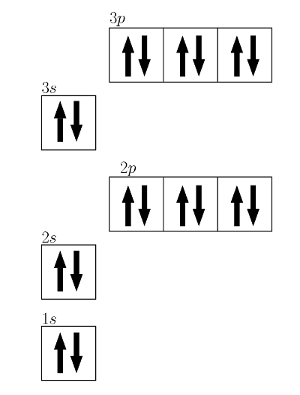Figure 4.12: An Aufbau diagram showing the electron configuration of argon

The spectroscopic electron configuration can be written in shorter form. This form is written as [noble gas]electrons, where the noble gas is the nearest one that occurs before the element. For example, magnesium can be represented as $$[\text{Ne}]3\text{s}^{2}$$ and carbon as $$[\text{H}]2\text{s}^{2}2\text{p}^{2}$$. This is known as the condensed electron configuration.

## Worked example 3: Aufbau diagrams and spectroscopic electron configuration

Give the electron configuration for nitrogen (N) and draw an Aufbau diagram.

### Give the number of electrons

Nitrogen has seven electrons.

### Place two electrons in the 1s orbital

We start by placing two electrons in the 1s orbital: $$1\text{s}^{2}$$.

Now we have five electrons left to place in orbitals.

### Place two electrons in the 2s orbital

We put two electrons in the 2s orbital: $$2\text{s}^{2}$$.

There are now three electrons to place in orbitals.

### Place three electrons in the 2p orbital

We place three electrons in the 2p orbital: $$2\text{p}^{3}$$.

The electron configuration is: $$1\text{s}^{2}2\text{s}^{2}2\text{p}^{3}$$. The Aufbau diagram is given in the step above.

#### Aufbau diagrams for ions

When a neutral atom loses an electron it becomes positively charged and we call it a cation. For example, sodium will lose one electron and become $$\text{Na}^{+}$$ or calcium will lose two electrons and become $$\text{Ca}^{2+}$$. In each of these cases, the outermost electron(s) will be lost.

When a neutral atom gains an electron it becomes negatively charged and we call it an anion. For example chlorine will gain one electron and become $$\text{Cl}^{-}$$ or oxygen will gain two electrons and become $$\text{O}^{2-}$$.

Aufbau diagrams and electron configurations can be done for cations and anions as well. The following worked example will show you how.

## Worked example 4: Aufbau diagram for an ion

Give the electron configuration for ($$\text{O}^{2-}$$) and draw an Aufbau diagram.

### Give the number of electrons

Oxygen has eight electrons. The oxygen anion has gained two electrons and so the total number of electrons is ten.

### Place two electrons in the 1s orbital

We start by placing two electrons in the 1s orbital: $$1\text{s}^{2}$$.

Now we have eight electrons left to place in orbitals.

### Place two electrons in the 2s orbital

We put two electrons in the 2s orbital: $$2\text{s}^{2}$$.

There are now six electrons to place in orbitals.

### Place six electrons in the 2p orbital

We place six electrons in the 2p orbital: $$2\text{p}^{6}$$.

The electron configuration is: $$1\text{s}^{2}2\text{s}^{2}2\text{p}^{6}$$. The Aufbau diagram is:

When we draw the orbitals we draw a shape that has a boundary (i.e. a closed shape). This represents the distance from the nucleus in which we are $$\text{95}\%$$ sure that we will find the electrons. In reality the electrons of an atom could be found any distance away from the nucleus.

### Orbital shapes (ESABJ)

Each of the orbitals has a different shape. The s orbitals are spherical and the p orbitals are dumbbell shaped.Figure 4.13: The orbital shapes. From left to right: an s orbital, a p orbital, the three p orbitals

### Core and valence electrons (ESABK)

Electrons in the outermost energy level of an atom are called valence electrons. The electrons that are in the energy shells closer to the nucleus are called core electrons. Core electrons are all the electrons in an atom, excluding the valence electrons. An element that has its valence energy level full is more stable and less likely to react than other elements with a valence energy level that is not full.

Valence electrons

The electrons in the outermost energy level of an atom.

Core electrons

All the electrons in an atom, excluding the valence electrons.

## Core and valence electrons

Textbook Exercise 4.4

Complete the following table:

 Element or Ion Electron configuration Core electrons Valence electrons Potassium ($$\text{K}$$) Helium ($$\text{He}$$) Oxygen ion ($$\text{O}^{2-}$$) Magnesium ion ($$\text{Mg}^{2+}$$)
Solution not yet available

### The importance of understanding electron configuration (ESABL)

By this stage, you may well be wondering why it is important for you to understand how electrons are arranged around the nucleus of an atom. Remember that during chemical reactions, when atoms come into contact with one another, it is the electrons of these atoms that will interact first. More specifically, it is the valence electrons of the atoms that will determine how they react with one another.

To take this a step further, an atom is at its most stable (and therefore unreactive) when all its orbitals are full. On the other hand, an atom is least stable (and therefore most reactive) when its valence electron orbitals are not full. This will make more sense when we go on to look at chemical bonding in a later chapter. To put it simply, the valence electrons are largely responsible for an element's chemical behaviour and elements that have the same number of valence electrons often have similar chemical properties.

The most stable configurations are the ones that have full energy levels. These configurations occur in the noble gases. The noble gases are very stable elements that do not react easily (if at all) with any other elements. This is due to the full energy levels. All elements would like to reach the most stable electron configurations, i.e. all elements want to be noble gases. This principle of stability is sometimes referred to as the octet rule. An octet is a set of 8, and the number of electrons in a full energy level is 8.

## Flame tests

### Aim

To determine what colour a metal cation will cause a flame to be.

### Apparatus

• Watch glass

• Bunsen burner

• methanol

• tooth picks (or skewer sticks)

• metal salts (e.g. $$\text{NaCl}$$, $$\text{CuCl}_{2}$$, $$\text{CaCl}_{2}$$, $$\text{KCl}$$, etc.)

• metal powders (e.g. copper, magnesium, zinc, iron, etc.)

Be careful when working with Bunsen burners as you can easily burn yourself. Make sure all scarves/loose clothing are securely tucked in and long hair is tied back. Ensure that you work in a well-ventilated space and that there is nothing flammable near the open flame.

### Method

For each salt or powder do the following:

1. Dip a clean tooth pick into the methanol

2. Dip the tooth pick into the salt or powder

3. Wave the tooth pick through the flame from the Bunsen burner. Do not hold the tooth pick in the flame, but rather wave it back and forth through the flame.

4. Observe what happens

### Results

Record your results in a table, listing the metal salt and the colour of the flame.

### Conclusion

You should have observed different colours for each of the metal salts and powders that you tested.

The above experiment on flame tests relates to the line emission spectra of the metals. These line emission spectra are a direct result of the arrangement of the electrons in metals. Each metal salt has a uniquely coloured flame.

## Energy diagrams and electrons

Textbook Exercise 4.5

Draw Aufbau diagrams to show the electron configuration of each of the following elements:

1. magnesium
2. potassium
3. sulfur
4. neon
5. nitrogen

Using the rules about drawing Aufbau diagrams we get the following.

1. Magnesium has 12 electrons. So we start with energy level 1 and put 2 electrons in it. Then we move to energy level two which has the 2s and the 2p orbitals. This level can hold 8 electrons. Adding up the total electrons used so far gives 10 electrons. So we must place two more electrons and these go into the third level.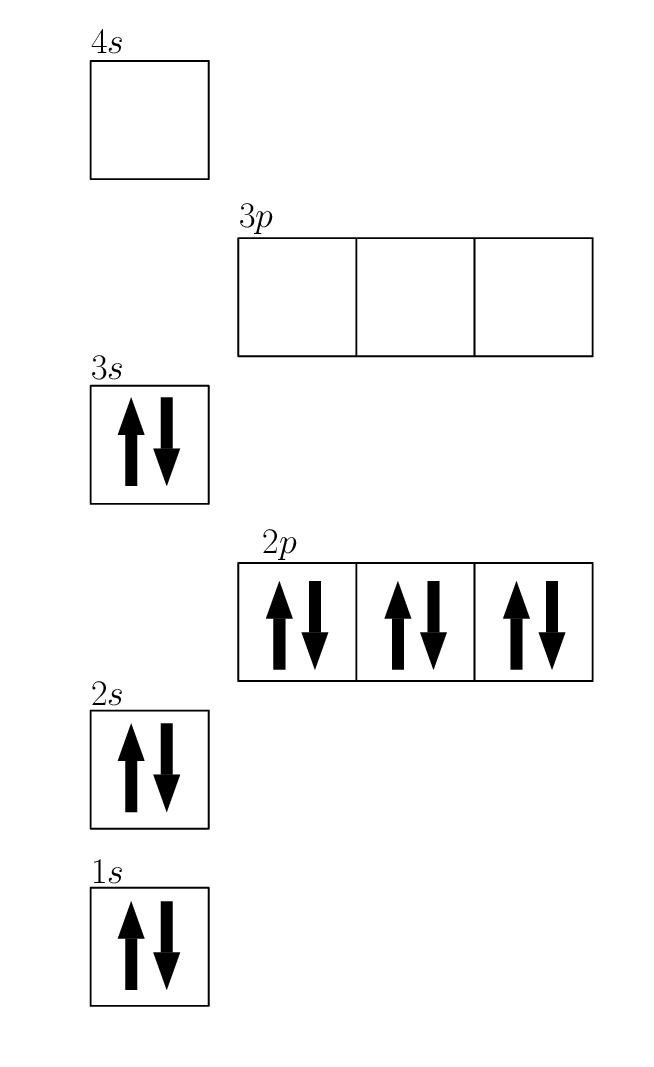2. Pottassium has 19 electrons. So we get: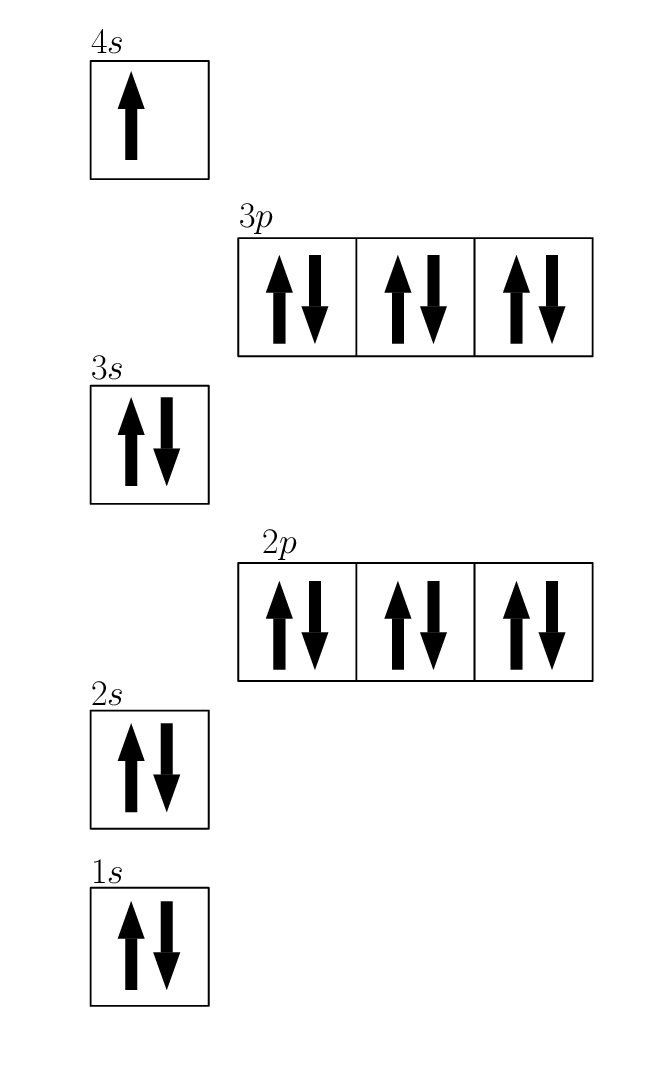3. Sulfur has 14 electrons so we get: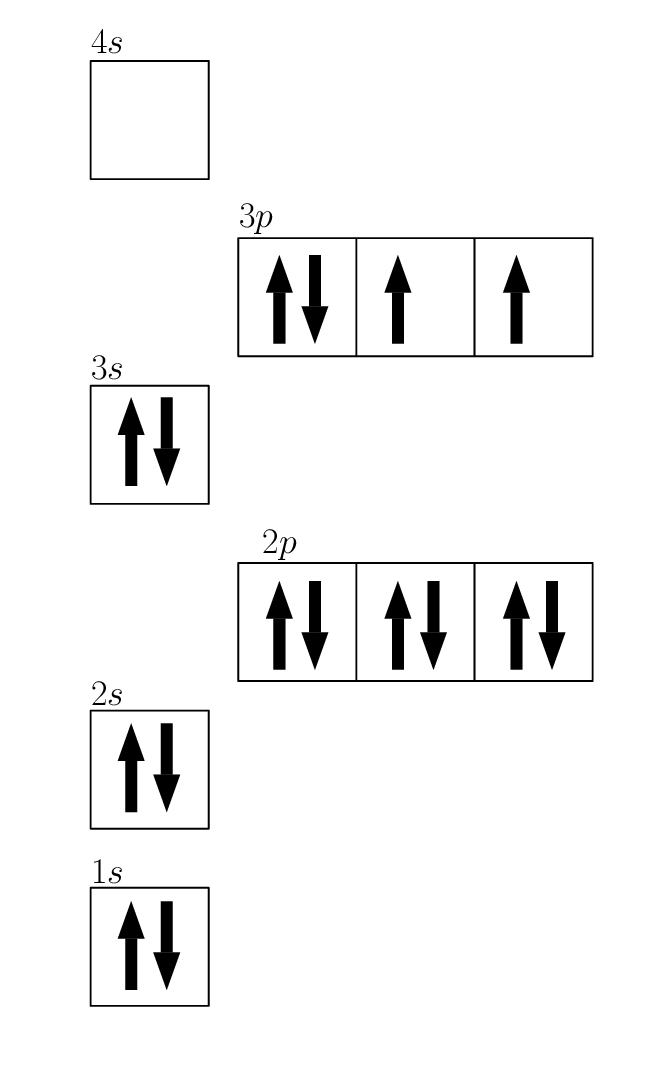4. Neon has 10 electrons so we get: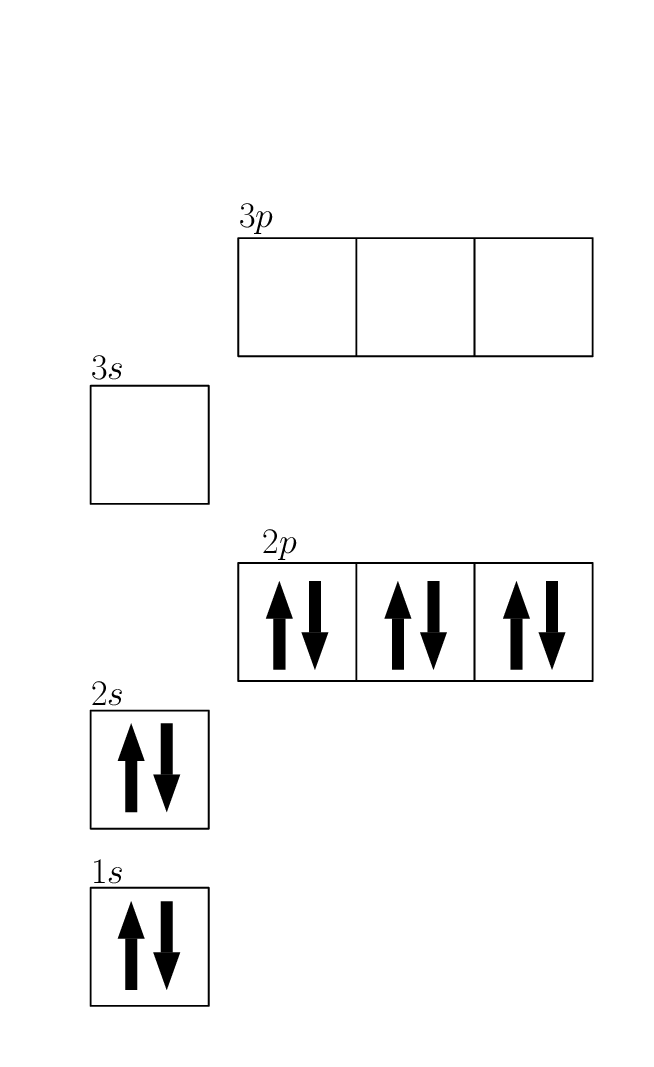5. Nitrogen has 7 electrons so we get: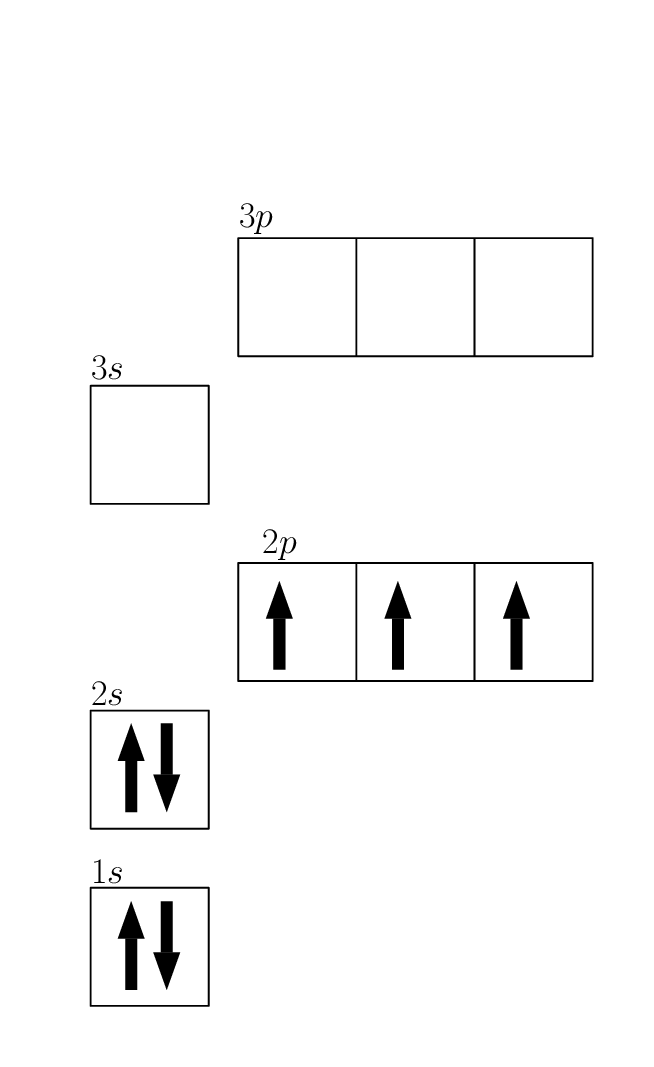Use the Aufbau diagrams you drew to help you complete the following table:

 Element No. of energy levels with electrons No. of electrons No. of valence electrons Electron configuration (standard notation) $$\text{Mg}$$ $$\text{K}$$ $$\text{S}$$ $$\text{Ne}$$ $$\text{N}$$

The energy levels are given by the numeral above the boxes, so 1, 2, 3 etc. The number of core electrons is all the electrons that are not in the outermost energy level. The number of valence electrons is the number of electrons in the outermost energy level. And the electron configuration is simply listing the energy levels together with the orbitals and the number of electrons in each orbital. So magnesium has electrons in 3 energy levels, the number of core electrons is 10, the number of valence electrons is 2 and the electron configuration is: $$1\text{s}^{2}2\text{s}^{2}2\text{p}^{6}3\text{s}^{2}$$. Filling in the table gives:

 Element No. of energy levels with electrons No. of electrons No. of valence electrons Electron configuration (standard notation) $$\text{Mg}$$ $$\text{3}$$ $$\text{10}$$ $$\text{2}$$ $$1\text{s}^{2}2\text{s}^{2}2\text{p}^{6}3\text{s}^{2}$$ $$\text{K}$$ $$\text{4}$$ $$\text{18}$$ $$\text{1}$$ $$1\text{s}^{2}2\text{s}^{2}2\text{p}^{6}3\text{s}^{2}3\text{p}^{6}4\text{s}^{1}$$ $$\text{S}$$ $$\text{3}$$ $$\text{10}$$ $$\text{6}$$ $$1\text{s}^{2}2\text{s}^{2}2\text{p}^{6}3\text{s}^{2}3\text{p}^{4}$$ $$\text{Ne}$$ $$\text{2}$$ $$\text{2}$$ $$\text{8}$$ $$1\text{s}^{2}2\text{s}^{2}2\text{p}^{6}$$ $$\text{N}$$ $$\text{2}$$ $$\text{2}$$ $$\text{5}$$ $$1\text{s}^{2}2\text{s}^{2}2\text{p}^{3}$$

Rank the elements used above in order of increasing reactivity. Give reasons for the order you give.

Solution not yet available.